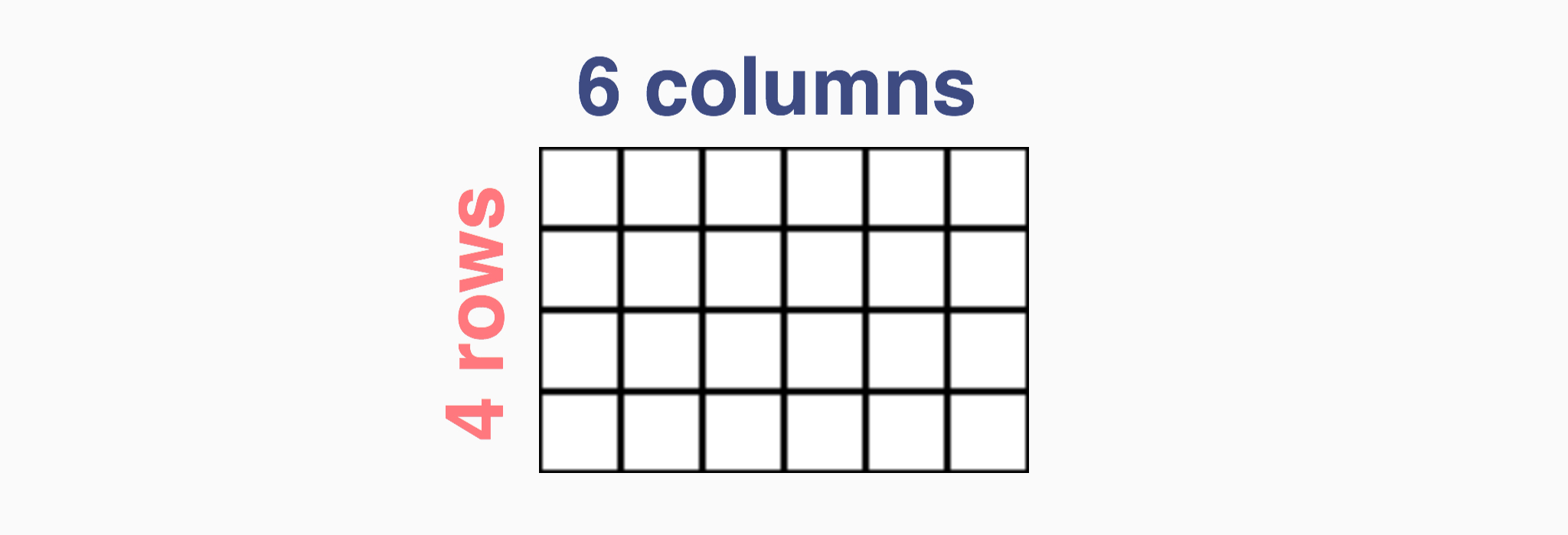Even or odd?
Name that shape
Arrays
Story Problems
100

Is 7 even or odd

7 is an odd number

100

3 corners and 3 sides

Triangle

100

Show me what a column looks like with your arm

it goes up and down

100

Royal and Jaylee went to Walmart. They wanted to buy a bag of candy that was \$3.00. If they had \$1.00 how much more money would they need to buy the candy?

\$2.00

100
Who is Ms. Crehan's favorite singer

Taylor Swift

200

Is 16 an even or an odd number

16 is an even number

200

4 corners and 4 sides

200

Show me what a row looks like with your arm

You arm will go side to side

200

Izabel and Jeremiah have some pattern blocks. If there are 10 pattern blocks all together how many can each of them get so they have the same number of pattern blocks?

5 pattern block for each

5+5=10

200

True or false (Jaylee and Zach you can't answer this one!)

Jaylee and Zach are birthday twins because they both are born on July 24th.

True!

300

When we decide whether a number is even or odd do we look at the hundreds place, tens place or ones place

We look at the ones place to decide if a number is even or odd

300

Our classroom door is an example of this kind of shape

Quadrilateral because it has 4 corners and 4 sides!

300

Antonio split a rectangle into equal size squares. Pick 3 correct answers about the diagram

I will draw the diagram on the board

A. The total number of equal sides is 5+5+5+5

B. The total number of equal sides is 4+4+4+4+4

C. The total number of this array is odd

D. The total number of this array is even

A.

B.

D.

300

Jeovany and Erick want to play a game at recess. There are 15 kids that are interested in playing with them. Can they make 2 teams with the same amount of players?

No they can't because 15 is an odd number.

300

J'Myah has a twin brother. What is his name?

Jamari

400

Is there an even or odd amount of students in our class

(hint there are 25 students in our class)

There is an odd amount of students in our class

400

5 corners and 5 sides

Pentagon

400

Ms. Crehan drew an array (I will show it to you on the board)

A. 4+4+4+4=16 total boxes

B. 4*4=16 total boxes

C. The amount of boxes is an odd number

C. The amount of boxes is an odd number

400

Isabelle and Gia have 223 mini erasers. Can they divide the erasers so both girls get the same number of mini erasers?

No they can't because 223 is an odd number.

400

True or false (Jeremiah and McKayla you can't answer this one!)

Jeremiah and McKayla have the same birthday that falls on this holiday

St. Patricks Day

March 17th

500

Look at this picture, is there an even or odd amount of boxes? How many boxes are there all together6+6+6+6=24

4+4+4+4+4+4=24

24 is an even number so there are an even number of boxes

500

8 corners and 8 sides

Octogon

500

Draw me an array with 4 columns and 3 rows

How many boxes are all together

I will draw the answer on the board

500

Koah and Oakley have some treats. Oakley has the same amount of treats as Koah. Pick 3 statements that could be true.

A. Oakley has an even number of treats

B. Koah has an odd number of treats

C. Koah has an even number of treats, Oakley has an odd number of treats.

D. All together Koah and Oakley have an even number of treats.

THIS IS ON THE TEST!

a, b, d

500

Ms. Crehan has tattoos what are her tattoos?

A rainbow on her wrist

A moon on her arm

Click to zoom